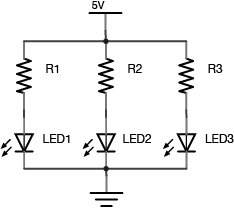# Week 2: Basic Electronics lab + quiz

#### Name and define are the three properties of an electrical circuit that we’ve described in class so far. How do they relate to each other?

Voltage is a measure of the difference in electrical energy between two points in a circuit.

Current is a measure of the level of the flow of electrons through a particular point in a circuit.

Resistance is a measure of a material’s ability to oppose the flow of electricity, and is measured in Ohms.

V = I x R

The higher the voltage, the more the potential energy has.

The amount of of energy flowing would be the current.

The resistance could help control the flow of energy and to avoid overpowering the energy.

#### Explain how electrical energy flows in a properly working circuit. What are the minimum necessary components? From where does it flow, and to where? What must happen to the energy as it moves through the circuit?

In the DC (Directing Current), the basic current consist of a LED lamp(Diodes), a pushbutton(switch)and a battery (power source). The battery is the source and the lamp is the load. The energy flow in one direction, from the source to the load, the energy will be converted into heat or other kinds of energy.*making a arduino basic circuit with LED and switch*

#### What is a short circuit? How does it differ from a properly working circuit?

When there is no load to convert electrical energy to other forms of energy is called short circuit. The power source feeds all of its power through the wires and back to itself, and the wires will melt, or the power source will blow up.

#### What are the following components? How are they commonly used in a circuit?

Resistors oppose the flow of electricity. They mostly used to control the flow of current. Current can move either way through a resistor. Resistors are measured by their resistance in ohms (Ω), often seen in kilohms (kΩ).

Potentiometer is a resistor that can change its resistance.

Diode allows the flow of electricity in one direction, and would block another way around. LED is one of the types of diodes that can emit light when current is flowing through them.

Transistor electrical switching devices, such as NPN, PNP and relay.

#### What formula would you use to calculate the value for the resistor in the following circuit? What is the ideal resistance value? What happens if the resistance is too low?

Ohm’s law:

I = V / R

so..R = V / I

= 150 Ω

5 V –> resistor (3V) –> LED (2V) –> 0V

5V – 2V / 0.02 A = 150 Ω

If resistance is too low, the more current it will get.

#### If you wanted to decrease the brightness of the LED in the circuit above, what change would you make?

Add a stronger resistor.

#### What are the first things you should check when troubleshooting any circuit?

Unplug the power source.

#### Identify the components that are in series below, and those that are in parallel:The components are in series in group of Resistor and LED, and operate parallel.
Resistors, Diodes, Ground, Power source.

When components are in series, the capacity through them is the same.
When components are in parallel, the voltage across them is the same.
When you put batteries in series the voltage adds up.
When you put batteries in parallel the capacity adds up.

Back to Top